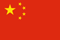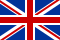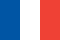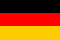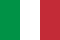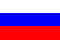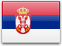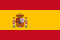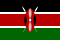Wednesday, 02 March 2011 03:15

## Statistical Methods

Written by
Rate this item
(0 votes)

There is much debate on the role of statistics in epidemiological research on causal relationships. In epidemiology, statistics is primarily a collection of methods for assessing data based on human (and also on animal) populations. In particular, statistics is a technique for the quantification and measurement of uncertain phenomena. All the scientific investigations which deal with non-deterministic, variable aspects of reality could benefit from statistical methodology. In epidemiology, variability is intrinsic to the unit of observation—a person is not a deterministic entity. While experimental designs would be improved in terms of better meeting the assumptions of statistics in terms of random variation, for ethical and practical reasons this approach is not too common. Instead, epidemiology is engaged in observational research which has associated with it both random and other sources of variability.

Statistical theory is concerned with how to control unstructured variability in the data in order to make valid inferences from empirical observations. Lacking any explanation for the variable behaviour of the phenomenon studied, statistics assumes it as random—that is, non-systematic deviations from some average state of nature (see Greenland 1990 for a criticism of these assumptions).

Science relies on empirical evidence to demonstrate whether its theoretical models of natural events have any validity. Indeed, the methods used from statistical theory determine the degree to which observations in the real world conform to the scientists’ view, in mathematical model form, of a phenomenon. Statistical methods, based in mathematics, have therefore to be carefully selected; there are plenty of examples about “how to lie with statistics”. Therefore, epidemiologists should be aware of the appropriateness of the techniques they apply to measure the risk of disease. In particular, great care is needed when interpreting both statistically significant and statistically non-significant results.

The first meaning of the word statistics relates to any summary quantity computed on a set of values. Descriptive indices or statistics such as the arithmetic average, the median or the mode, are widely used to summarize the information in a series of observations. Historically, these summary descriptors were used for administrative purposes by states, and therefore they were named statistics. In epidemiology, statistics that are commonly seen derive from the comparisons inherent to the nature of epidemiology, which asks questions such as: “Is one population at greater risk of disease than another?” In making such comparisons, the relative risk is a popular measure of the strength of association between an individual characteristic and the probability of becoming ill, and it is most commonly applied in aetiological research; attributable risk is also a measure of association between individual characteristics and disease occurrence, but it emphasizes the gain in terms of number of cases spared by an intervention which removes the factor in question—it is mostly applied in public health and preventive medicine.

The second meaning of the word statistics relates to the collection of techniques and the underlying theory of statistical inference. This is a particular form of inductive logic which specifies the rules for obtaining a valid generalization from a particular set of empirical observations. This generalization would be valid provided some assumptions are met. This is the second way in which an uneducated use of statistics can deceive us: in observational epidemiology, it is very difficult to be sure of the assumptions implied by statistical techniques. Therefore, sensitivity analysis and robust estimators should be companions of any correctly conducted data analysis. Final conclusions also should be based on overall knowledge, and they should not rely exclusively on the findings from statistical hypothesis testing.

Definitions

A statistical unit is the element on which the empirical observations are made. It could be a person, a biological specimen or a piece of raw material to be analysed. Usually the statistical units are independently chosen by the researcher, but sometimes more complex designs can be set up. For example, in longitudinal studies, a series of determinations is made on a collection of persons over time; the statistical units in this study are the set of determinations, which are not independent, but structured by their respective connections to each person being studied. Lack of independence or correlation among statistical units deserves special attention in statistical analysis.

A variable is an individual characteristic measured on a given statistical unit. It should be contrasted with a constant, a fixed individual characteristic—for example, in a study on human beings, having a head or a thorax are constants, while the gender of a single member of the study is a variable.

Variables are evaluated using different scales of measurement. The first distinction is between qualitative and quantitative scales. Qualitative variables provide different modalities or categories. If each modality cannot be ranked or ordered in relation to others—for example, hair colour, or gender modalities—we denote the variable as nominal. If the categories can be ordered—like degree of severity of an illness—the variable is called ordinal. When a variable consists of a numeric value, we say that the scale is quantitative. A discrete scale denotes that the variable can assume only some definite values—for example, integer values for the number of cases of disease. A continuous scale is used for those measures which result in real numbers. Continuous scales are said to be interval scales when the null value has a purely conventional meaning. That is, a value of zero does not mean zero quantity—for example, a temperature of zero degrees Celsius does not mean zero thermal energy. In this instance, only differences among values make sense (this is the reason for the term “interval” scale). A real null value denotes a ratio scale. For a variable measured on that scale, ratios of values also make sense: indeed, a twofold ratio means double the quantity. For example, to say that a body has a temperature two times greater than a second body means that it has two times the thermal energy of the second body, provided that the temperature is measured on a ratio scale (e.g., in Kelvin degrees). The set of permissible values for a given variable is called the domain of the variable.

Statistical Paradigms

Statistics deals with the way to generalize from a set of particular observations. This set of empirical measurements is called a sample. From a sample, we calculate some descriptive statistics in order to summarize the information collected.

The basic information that is generally required in order to characterize a set of measures relates to its central tendency and to its variability. The choice between several alternatives depends on the scale used to measure a phenomenon and on the purposes for which the statistics are computed. In table 1 different measures of central tendency and variability (or, dispersion) are described and associated with the appropriate scale of measurement.

Table 1. Indices of central tendency and dispersion by scale of measurement

 Scale of measurement Qualitative Quantitative Indices Definition Nominal Ordinal Interval/ratio Arithmetic mean Sum of the observed values divided by the total number of observations x Median Midpoint value of the observed distribution x x Mode Most frequent value x x x Range Lowest and highest values of the distribution x x Variance Sum of the squared difference of each value from the mean divided by the total number of observations minus 1 x

The descriptive statistics computed are called estimates when we use them as a substitute for the analogous quantity of the population from which the sample has been selected. The population counterparts of the estimates are constants called parameters. Estimates of the same parameter can be obtained using different statistical methods. An estimate should be both valid and precise.

The population-sample paradigm implies that validity can be assured by the way the sample is selected from the population. Random or probabilistic sampling is the usual strategy: if each member of the population has the same probability of being included in the sample, then, on average, our sample should be representative of the population and, moreover, any deviation from our expectation could be explained by chance. The probability of a given deviation from our expectation also can be computed, provided that random sampling has been performed. The same kind of reasoning applies to the estimates calculated for our sample with regard to the population parameters. We take, for example, the arithmetic average from our sample as an estimate of the mean value for the population. Any difference, if it exists, between the sample average and the population mean is attributed to random fluctuations in the process of selection of the members included in the sample. We can calculate the probability of any value of this difference, provided the sample was randomly selected. If the deviation between the sample estimate and the population parameter cannot be explained by chance, the estimate is said to be biased. The design of the observation or experiment provides validity to the estimates and the fundamental statistical paradigm is that of random sampling.

In medicine, a second paradigm is adopted when a comparison among different groups is the aim of the study. A typical example is the controlled clinical trial: a set of patients with similar characteristics is selected on the basis of pre-defined criteria. No concern for representativeness is made at this stage. Each patient enrolled in the trial is assigned by a random procedure to the treatment group—which will receive standard therapy plus the new drug to be evaluated—or to the control group—receiving the standard therapy and a placebo. In this design, the random allocation of the patients to each group replaces the random selection of members of the sample. The estimate of the difference between the two groups can be assessed statistically because, under the hypothesis of no efficacy of the new drug, we can calculate the probability of any non-zero difference.

In epidemiology, we lack the possibility of assembling randomly exposed and non-exposed groups of people. In this case, we still can use statistical methods, as if the groups analysed had been randomly selected or allocated. The correctness of this assumption relies mainly on the study design. This point is particularly important and underscores the importance of epidemiological study design over statistical techniques in biomedical research.

Signal and Noise

The term random variable refers to a variable for which a defined probability is associated with each value it can assume. The theoretical models for the distribution of the probability of a random variable are population models. The sample counterparts are represented by the sample frequency distribution. This is a useful way to report a set of data; it consists of a Cartesian plane with the variable of interest along the horizontal axis and the frequency or relative frequency along the vertical axis. A graphic display allows us to readily see what is (are) the most frequent value(s) and how the distribution is concentrated around certain central values like the arithmetic average.

For the random variables and their probability distributions, we use the terms parameters, mean expected value (instead of arithmetic average) and variance. These theoretical models describe the variability in a given phenomenon. In information theory, the signal is represented by the central tendency (for example, the mean value), while the noise is measured by a dispersion index (such as the variance).

To illustrate statistical inference, we will use the binomial model. In the sections which follow, the concepts of point estimates and confidence intervals, tests of hypotheses and probability of erroneous decisions, and power of a study will be introduced.

Table 2. Possible outcomes of a binomial experiment (yes = 1, no = 0) and their probabilities (n = 3)

 Worker Probability A B C 0 0 01 0 00 1 00 0 10 1 11 0 11 1 01 1 1An Example: The Binomial Distribution

In biomedical research and epidemiology, the most important model of stochastic variation is the binomial distribution. It relies on the fact that most phenomena behave as a nominal variable with only two categories: for example, the presence/absence of disease: alive/dead, or recovered/ill. In such circumstances, we are interested in the probability of success—that is, in the event of interest (e.g., presence of disease, alive or recovery)—and in the factors or variables that can alter it. Let us consider n = 3 workers, and suppose that we are interested in the probability, p, of having a visual impairment (yes/no). The result of our observation could be the possible outcomes in table 2.

Table 3. Possible outcomes of a binomial experiment (yes = 1, no = 0) and their probabilities (n = 3)

 Number of successes Probability 0123The probability of any of these event combinations is easily obtained by considering p, the (individual) probability of success, constant for each subject and independent from other outcomes. Since we are interested in the total number of successes and not in a specific ordered sequence, we can rearrange the table as follows (see table 3) and, in general, express the probability of x successes P(x) as:where x is the number of successes and the notation x! denotes the factorial of x, i.e., x! = x×(x–1)×(x–2)…×1.

When we consider the event “being/not being ill”, the individual probability,refers to the state in which the subject is presumed; in epidemiology, this probability is called “prevalence”. To estimate p, we use the sample proportion:

p = x/n

with variance:In an hypothetical infinite series of replicated samples of the same size n, we would obtain different sample proportions p = x/n, with probabilities given by the binomial formula. The “true” value ofis estimated by each sample proportion, and a confidence interval for p, that is, the set of likely values for p, given the observed data and a pre-defined level of confidence (say 95%), is estimated from the binomial distribution as the set of values for p which gives a probability of x greater than a pre-specified value (say 2.5%). For a hypothetical experiment in which we observed x = 15 successes in n = 30 trials, the estimated probability of success is:

p = x/n = 15/30 = 0.5

Table 4. Binomial distribution. Probabilities for different values offor x = 15 successes in n = 30 trialsProbability 0.200 0.0002 0.300 0.0116 0.334 0.025 0.400 0.078 0.500 0.144 0.600 0.078 0.666 0.025 0.700 0.0116

The 95% confidence interval for p, obtained from table 4, is 0.334 – 0.666. Each entry of the table shows the probability of x = 15 successes in n = 30 trials computed with the binomial formula; for example, for= 0.30, we obtain from:For n large and p close to 0.5 we can use an approximation based on the Gaussian distribution:where za /2 denotes the value of the standard Gaussian distribution for a probability

P (|z| ³ za /2) = a/2;

1 – a being the chosen confidence level. For the example considered,= 15/30 = 0.5; n = 30 and from the standard Gaussian table z0.025 = 1.96. The 95% confidence interval results in the set of values 0.321 – 0.679, obtained by substituting p = 0.5, n = 30, and z0.025 = 1.96 into the above equation for the Gaussian distribution. Note that these values are close to the exact values computed before.

Statistical tests of hypotheses comprise a decision procedure about the value of a population parameter. Suppose, in the previous example, that we want to address the proposition that there is an elevated risk of visual impairment among workers of a given plant. The scientific hypothesis to be tested by our empirical observations then is “there is an elevated risk of visual impairment among workers of a given plant”. Statisticians demonstrate such hypotheses by falsifying the complementary hypothesis “there is no elevation of the risk of visual impairment”. This follows the mathematical demonstration per absurdum and, instead of verifying an assertion, empirical evidence is used only to falsify it. The statistical hypothesis is called the null hypothesis. The second step involves specifying a value for the parameter of that probability distribution used to model the variability in the observations. In our examples, since the phenomenon is binary (i.e., presence/absence of visual impairment), we choose the binomial distribution with parameter p, the probability of visual impairment. The null hypothesis asserts that= 0.25, say. This value is chosen from the collection of knowledge about the topic and a priori knowledge of the usual prevalence of visual impairment in non-exposed (i.e., non-worker) populations. Suppose our data produced an estimate= 0.50, from the 30 workers examined.

Can we reject the null hypothesis?

If yes, in favour of what alternative hypothesis?

We specify an alternative hypothesis as a candidate should the evidence dictate that the null hypothesis be rejected. Non-directional (two-sided) alternative hypotheses state that the population parameter is different from the value stated in the null hypothesis; directional (one-sided) alternative hypotheses state that the population parameter is greater (or lesser) than the null value.

Table 5. Binomial distribution. Probabilities of success for= 0.25 in n = 30 trials

 X Probability Cumulative probability 0 0.0002 0.0002 1 0.0018 0.0020 2 0.0086 0.0106 3 0.0269 0.0374 4 0.0604 0.0979 5 0.1047 0.2026 6 0.1455 0.3481 7 0.1662 0.5143 8 0.1593 0.6736 9 0.1298 0.8034 10 0.0909 0.8943 11 0.0551 0.9493 12 0.0291 0.9784 13 0.0134 0.9918 14 0.0054 0.9973 15 0.0019 0.9992 16 0.0006 0.9998 17 0.0002 1.0000 . . . 30 0.0000 1.0000

Under the null hypothesis, we can calculate the probability distribution of the results of our example. Table 5 shows, for= 0.25 and n = 30, the probabilities (see equation (1)) and the cumulative probabilities:From this table, we obtain the probability of having x ³15 workers with visual impairment

P(x ³15) = 1 – P(x <15) = 1 – 0.9992 = 0.0008

This means that it is highly improbable that we would observe 15 or more workers with visual impairment if they experienced the prevalence of disease of the non-exposed populations. Therefore, we could reject the null hypothesis and affirm that there is a higher prevalence of visual impairment in the population of workers that was studied.

When n×p ³ 5 and n×(1-) ³ 5, we can use the Gaussian approximation:From the table of the standard Gaussian distribution we obtain:

P(|z|>2.95) = 0.0008

in close agreement with the exact results. From this approximation we can see that the basic structure of a statistical test of hypothesis consists of the ratio of signal to noise. In our case, the signal is (p), the observed deviation from the null hypothesis, while the noise is the standard deviation of P:The greater the ratio, the lesser the probability of the null value.

In making decisions about statistical hypotheses, we can incur two kinds of errors: a type I error, rejection of the null hypothesis when it is true; or a type II error, acceptance of the null hypothesis when it is false. The probability level, or p-value, is the probability of a type I error, denoted by the Greek letter a. This is calculated from the probability distribution of the observations under the null hypothesis. It is customary to predefine an a-error level (e.g., 5%, 1%) and reject the null hypothesis when the result of our observation has a probability equal to or less than this so-called critical level.

The probability of a type II error is denoted by the Greek letter β. To calculate it, we need to specify, in the alternative hypothesis, α value for the parameter to be tested (in our example, α value for). Generic alternative hypotheses (different from, greater than, less than) are not useful. In practice, the β-value for a set of alternative hypotheses is of interest, or its complement, which is called the statistical power of the test. For example, fixing the α-error value at 5%, from table 5, we find:

P(x ³12) <0.05

under the null hypothesis= 0.25. If we were to observe at least x = 12 successes, we would reject the null hypothesis. The corresponding β values and the power for x = 12 are given by table 6.

Table 6. Type II error and power for x = 12, n = 30, α = 0.05β Power 0.30 0.9155 0.0845 0.35 0.7802 0.2198 0.40 0.5785 0.4215 0.45 0.3592 0.6408 0.50 0.1808 0.8192 0.55 0.0714 0.9286

In this case our data cannot discriminate whetheris greater than the null value of 0.25 but less than 0.50, because the power of the study is too low (<80%) for those values of<0.50—that is, the sensitivity of our study is 8% for= 0.3, 22% for= 0.35,…, 64% for= 0.45.

The only way to achieve a lower β, or a higher level of power, would be to increase the size of the study. For example, in table 7 we report β and power for n = 40; as expected, we should be able to detect avalue greater than 0.40.

Table 7. Type II error and power for x = 12, n = 40, α = 0.05β Power 0.30 0.5772 0.4228 0.35 0.3143 0.6857 0.40 0.1285 0.8715 0.45 0.0386 0.8614 0.50 0.0083 0.9917 0.55 0.0012 0.9988

Study design is based on careful scrutiny of the set of alternative hypotheses which deserve consideration and guarantee power to the study providing an adequate sample size.

In the epidemiological literature, the relevance of providing reliable risk estimates has been emphasized. Therefore, it is more important to report confidence intervals (either 95% or 90%) than a p-value of a test of a hypothesis. Following the same kind of reasoning, attention should be given to the interpretation of results from small-sized studies: because of low power, even intermediate effects could be undetected and, on the other hand, effects of great magnitude might not be replicated subsequently.

Advanced Methods

The degree of complexity of the statistical methods used in the occupational medicine context has been growing over the last few years. Major developments can be found in the area of statistical modelling. The Nelder and Wedderburn family of non-Gaussian models (Generalized Linear Models) has been one of the most striking contributions to the increase of knowledge in areas such as occupational epidemiology, where the relevant response variables are binary (e.g., survival/death) or counts (e.g., number of industrial accidents).

This was the starting point for an extensive application of regression models as an alternative to the more traditional types of analysis based on contingency tables (simple and stratified analysis). Poisson, Cox and logistic regression are now routinely used for the analysis of longitudinal and case-control studies, respectively. These models are the counterpart of linear regression for categorical response variables and have the elegant feature of providing directly the relevant epidemiological measure of association. For example, the coefficients of Poisson regression are the logarithm of the rate ratios, while those of logistic regression are the log of the odds ratios.

Taking this as a benchmark, further developments in the area of statistical modelling have taken two main directions: models for repeated categorical measures and models which extend the Generalized Linear Models (Generalized Additive Models). In both instances, the aims are focused on increasing the flexibility of the statistical tools in order to cope with more complex problems arising from reality. Repeated measures models are needed in many occupational studies where the units of analysis are at the sub-individual level. For example:

1. The study of the effect of working conditions on carpal tunnel syndrome has to consider both hands of a person, which are not independent from one other.
2. The analysis of time trends of environmental pollutants and their effect on children’s respiratory systems can be evaluated using extremely flexible models since the exact functional form of the dose-response relationship is difficult to obtain.

A parallel and probably faster development has been seen in the context of Bayesian statistics. The practical barrier of using Bayesian methods collapsed after the introduction of computer-intensive methods. Monte Carlo procedures such as Gibbs sampling schemes have allowed us to avoid the need for numerical integration for computing the posterior distributions which represented the most challenging feature of Bayesian methods. The number of applications of Bayesian models in real and complex problems have found increasing space in applied journals. For example, geographical analyses and ecological correlations at the small area level and AIDS prediction models are more and more often tackled using Bayesian approaches. These developments are welcomed because they represent not only an increase in the number of alternative statistical solutions which could be employed in the analysis of epidemiological data, but also because the Bayesian approach can be considered a more sound strategy.

Back

### Additional Info

Read 3829 times Last modified on Thursday, 13 October 2011 20:24### Latest from ILO Content Manager

" DISCLAIMER: The ILO does not take responsibility for content presented on this web portal that is presented in any language other than English, which is the language used for the initial production and peer-review of original content. Certain statistics have not been updated since the production of the 4th edition of the Encyclopaedia (1998)."

## Contents

Part I. The Body
Part II. Health Care
Part III. Management & Policy
Part IV. Tools and Approaches
Part V. Psychosocial and Organizational Factors
Part VI. General Hazards
Part VII. The Environment
Part VIII. Accidents and Safety Management
Part IX. Chemicals
Part X. Industries Based on Biological Resources
Part XI. Industries Based on Natural Resources
Part XII. Chemical Industries
Part XIII. Manufacturing Industries
Part XIV. Textile and Apparel Industries
Part XV. Transport Industries
Part XVI. Construction
Part XVII. Services and Trade
Part XVIII. Guides

## Epidemiology and Statistics References

Ahlbom, A. 1984. Criteria of causal association in epidemiology. In Health, Disease, and Causal Explanations in Medicine, edited by L Nordenfelt and BIB Lindahl. Dordrecht: D Reidel.

American Conference of Government Industrial Hygienists (ACGIH). 1991. Exposure Assessment for Epidemiology and Hazard Control, edited by SM Rappaport and TJ Smith. Chelsea, Mich.:Lewis.

Armstrong, BK, E White, and R Saracci. 1992. Principles of Exposure Measurement in Epidemiology. Oxford: Oxford Univ. Press.

Ashford, NA, CI Spadafor, DB Hattis, and CC Caldart. 1990. Monitoring the Worker for Exposure and Disease. Baltimore: Johns Hopkins Univ. Press.

Axelson, O. 1978. Aspects on confounding in occupational health epidemiology. Scand J Work Environ Health 4:85-89.

—. 1994. Some recent developments in occupational epidemiology. Scand J Work Environ Health 20 (Special issue):9-18.

Ayrton-Paris, JA. 1822. Pharmacologia.

Babbie, E. 1992. The Practice of Social Research. Belmont, Calif.: Wadsworth.

Beauchamp, TL, RR Cook, WE Fayerweather, GK Raabe, WE Thar, SR Cowles, and GH Spivey. 1991. Ethical Guidelines for Epidemiologists. J Clin Epidemiol 44 Suppl. I:151S-169S.

Bell, B. 1876. Paraffin epithelioma of the scrotum. Edinburgh Med J 22:135.

Blondin, O and C Viau. 1992. Benzo(a)pyrene-blood protein adducts in wild woodchucks used as biological sentinels of environmental polycyclic aromatic hydrocarbons contamination. Arch Environ Contam Toxicol 23:310-315.

Buck, C. 1975. Popper’s philosophy for epidemiologists. Int J Epidemiol 4:159-168.

Case, RAM and ME Hosker. 1954. Tumour on the urinary bladder as an occupational disease in the rubber industry in England and Wales. Brit J Prevent Soc Med 8:39-50.

Checkoway, H, NE Pearce, and DJ Crawford-Brown. 1989. Research Methods in Occupational Epidemiology. New York: Oxford Univ. Press.

Clayson, DB. 1962. Chemical Carcinogenesis. London: JA Churchill.

Clayton, D. 1992. Teaching statistical methods in epidemiology. In Epidemiology. What You Should Know and What You Could Do, edited by J Olsen and D Trichopoulos. Oxford: Oxford Univ. Press.

Clayton, D and M Hills. 1993. Statistical Models in Epidemiology. New York: Oxford Univ. Press.

Cornfield, J. 1954. Statistical relationships and proof in medicine. Am Stat 8:19-21.

Council for International Organizations of Medical Sciences (CIOMS). 1991. International Guidelines for Ethical Review of Epidemiologic Studies. Geneva: CIOMS.

Czaja, R and J Blair. 1996. Designing Surveys. Thousand Oaks, Calif: Pine Forge Press.

Doll, R. 1952. The causes of death among gas-workers with special reference to cancer of the lung. Brit J Ind Med 9:180-185.

—. 1955. Mortality from lung cancer in asbestos workers. Brit J Ind Med 12:81-86.

Droz, PO and MM Wu. 1991. Biological monitoring strategies. In Exposure Assessment for Epidemiology and Hazard Control, edited by SM Rappaport and TJ Smith. Chelsea, Mich.: Lewis.

Gamble, J and R Spirtas. 1976. Job classification and utilization of complete work histories in occupational epidemiology. J Med 18:399-404.

Gardner, MJ and DG Altman. 1989. Statistics With Confidence. Confidence Intervals and Statistical Guidelines. London: BMJ Publishing House.

Garfinkel, L. 1984. Classics in oncology; E. Cuyler Hammond, ScD. Ca-Cancer Journal for Clinicians. 38(1): 23-27

Giere, RN. 1979. Understanding Scientific Reasoning. New York: Holt Rinehart & Winston.

Glickman, LT. 1993. Natural exposure studies in pet animals: Sentinels for environmental carcinogens. Vet Can Soc Newslttr 17:5-7.

Glickman, LT, LM Domanski, TG Maguire, RR Dubielzig, and A Churg. 1983. Mesothelioma in pet dogs associated with exposure of their owners to asbestos. Environmental Research 32:305-313.

Gloyne, SR. 1935. Two cases of squamous carcinoma of the lung occurring in asbestosis. Tubercle 17:5-10.

—. 1951. Pneumoconiosis: Histological survey of necropsy material in 1,205 cases. Lancet 1:810-814.

Greenland, S. 1987. Quantitative methods in the review of epidemiological literature. Epidemiol Rev 9:1-30.

—. 1990. Randomization, statistics, and causal inference. Epidemiology 1:421-429.

Harting, FH and W Hesse. 1879. Der Lungenkrebs, die bergkrankheit in den Schneeberger Gruben. Vierteljahrsschr Gerichtl Med Offentl Gesundheitswesen CAPS 30:296-307.

Hayes, RB, JW Raatgever, A de Bruyn, and M Gerin. 1986. Cancer of the nasal cavity and paranasal sinuses, and formaldehyde exposure. Int J Cancer 37:487-492.

Hayes, HM, RE Tarone, HW Casey, and DL Huxsoll. 1990. Excess of seminomas observed in Vietnam service US military working dogs. J Natl Cancer Inst 82:1042-1046.

Hernberg, S. 1992. Introduction to Occupational Epidemiology. Chelsea, Mich.: Lewis.
Hill, AB. 1965. The environment and disease: Association or causation? Proc Royal Soc Med 58:295-300.

Hume, D. 1978. A Treatise of Human Nature. Oxford: Clarendon Press.

Hungerford, LL, HL Trammel, and JM Clark. 1995. The potential utility of animal poisoning data to identify human exposure to environmental toxins. Vet Hum Toxicol 37:158-162.

Jeyaratnam, J. 1994. Transfer of hazardous industries. In Occupational Cancer in Developing Countries, edited by NE Pearce, E Matos, H Vainio, P Boffetta, and M Kogevinas. Lyon: IARC.

Karhausen, LR. 1995. The poverty of Popperian epidemiology. Int J Epidemiol 24:869-874.

Kogevinas, M, P Boffetta, and N Pearce. 1994. Occupational exposure to carcinogens in developing countries. In Occupational Cancer in Developing Countries, edited by NE Pearce, E Matos, H Vainio, P Boffetta, and M Kogevinas. Lyon: IARC.

LaDou, J. 1991. Deadly migration. Tech Rev 7:47-53.

Laurell, AC, M Noriega, S Martinez, and J Villegas. 1992. Participatory research on workers’ health. Soc Sci Med 34:603-613.

Lilienfeld, AM and DE Lilienfeld. 1979. A century of case-control studies: progress? Chron Dis 32:5-13.

Loewenson, R and M Biocca. 1995. Participatory approaches in occupational health research. Med Lavoro 86:263-271.

Lynch, KM and WA Smith. 1935. Pulmonary asbestosis. III Carcinoma of lung in asbestos-silicosis. Am J Cancer 24:56-64.

Maclure, M. 1985. Popperian refutation in epidemiolgy. Am J Epidemiol 121:343-350.

—. 1988. Refutation in epidemiology: Why else not? In Causal Inference, edited by KJ Rothman. Chestnut Hill, Mass.: Epidemiology Resources.

Martin, SW, AH Meek, and P Willeberg. 1987. Veterinary Epidemiology. Des Moines: Iowa State Univ. Press.

McMichael, AJ. 1994. Invited commentary -"Molecular epidemiology": New pathway or new travelling companion? Am J Epidemiol 140:1-11.

Merletti, F and P Comba. 1992. Occupational epidemiology. In Teaching Epidemiology. What You Should Know and What You Could Do, edited by J Olsen and D Trichopoulos. Oxford: Oxford Univ. Press.

Miettinen, OS. 1985. Theoretical Epidemiology. Principles of Occurrence Research in Medicine. New York: John Wiley & Sons.

Newell, KW, AD Ross, and RM Renner. 1984. Phenoxy and picolinic acid herbicides and small-intestinal adenocarcinoma in sheep. Lancet 2:1301-1305.

Olsen, J, F Merletti, D Snashall, and K Vuylsteek. 1991. Searching for Causes of Work-Related Diseases. An Introduction to Epidemiology At the Work Site. Oxford: Oxford Medical Publications, Oxford Univ. Press.

Pearce, N. 1992. Methodological problems of time-related variables in occupational cohort studies. Rev Epidmiol Med Soc Santé Publ 40 Suppl: 43-54.

—. 1996. Traditional epidemiology, modern epidemiology and public health. Am J Public Health 86(5): 678-683.

Pearce, N, E Matos, H Vainio, P Boffetta, and M Kogevinas. 1994. Occupational cancer in developing countries. IARC Scientific Publications, no. 129. Lyon: IARC.

Pearce, N, S De Sanjose, P Boffetta, M Kogevinas, R Saracci, and D Savitz. 1995. Limitations of biomarkers of exposure in cancer epidemiology. Epidemiology 6:190-194.

Poole, C. 1987. Beyond the confidence interval. Am J Public Health 77:195-199.

Pott, P. 1775. Chirurgical Observations. London: Hawes, Clarke & Collins.

Proceedings of the Conference on Retrospective Assessment of Occupational Exposures in Epidemiology, Lyon, 13-15 April, 1994. 1995. Lyon: IARC .

Ramazzini, B. 1705. De Morbis Artificum Diatriva. Typis Antonii Capponi. Mutinae, MDCC. London: Andrew Bell & Others.

Rappaport, SM, H Kromhout, and E Symanski. 1993. Variation of exposure between workers in homogeneous exposure groups. Am Ind Hyg Assoc J 54(11):654-662.

Reif, JS, KS Lower, and GK Ogilvie. 1995. Residential exposure to magnetic fields and risk of canine lymphoma. Am J Epidemiol 141:3-17.

Reynolds, PM, JS Reif, HS Ramsdell, and JD Tessari. 1994. Canine exposure to herbicide-treated lawns and urinary excretion of 2,4-dichlorophenoxyacetic acid. Canc Epidem, Biomark and Prevention 3:233-237.

Robins, JM, D Blevins, G Ritter, and M Wulfsohn. 1992. G-estimation of the effect of prophylaxis therapy for pneumocystis carinii pneumonia on the survival of Aids patients. Epidemiology 3:319-336.

Rothman, KJ. 1986. Modern Epidemiology. Boston: Little, Brown & Co.

Saracci, R. 1995. Epidemiology: Yesterday, today, tomorrow. In Lectures and Current Topics in Epidemiology. Florence: European Educational Programme in Epidemiology.

Schaffner, KF. 1993. Discovery and Explanation in Biology and Medicine. Chicago: Univ. of Chicago Press.

Schlesselman, JJ. 1987. “Proof” of cause and effect in epidemiologic studies: Criteria for judgement. Prevent Med 16:195-210.

Schulte, P. 1989. Interpretation and communcication of the results of medical field investigations. J Occup Med 31:5889-5894.

Schulte, PA, WL Boal, JM Friedland, JT Walker, LB Connally, LF Mazzuckelli, and LJ Fine. 1993. Methodological issues in risk communications to workers. Am J Ind Med 23:3-9.

Schwabe, CW. 1993. The current epidemiological revolution in veterinary medicine. Part II. Prevent Vet Med 18:3-16.

Seidman, H, IJ Selikoff, and EC Hammond. 1979. Short-term asbestos work exposure and long-term observation. Ann NY Acad Sci 330:61-89.

Selikoff, IJ, EC Hammond, and J Churg. 1968. Asbestos exposure, smoking and neoplasia. JAMA 204:106-112.

—. 1964. Asbestos exposure and neoplasia. JAMA 188, 22-26.

Siemiatycki, J, L Richardson, M Gérin, M Goldberg, R Dewar, M Désy, S Campbell, and S Wacholder. 1986. Associations between several sites of cancer and nine organic dusts: Results from an hypothesis-generating case-control study in Montreal, 1979-1983. Am J Epidemiol 123:235-249.

Simonato, L. 1986. Occupational cancer risk in developing countries and priorities for epidemiological research. Presented at International Symposium On Health and Environment in Developing Countries, Haicco.

Smith, TJ. 1987. Exposure asssessment for occupational epidemiology. Am J Ind Med 12:249-268.

Soskolne, CL. 1985. Epidemiological research, interest groups, and the review process. J Publ Health Policy 6(2):173-184.

—. 1989. Epidemiology: Questions of science, ethics, morality and law. Am J Epidemiol 129(1):1-18.

—. 1993. Introduction to misconduct in science and scientific duties. J Expos Anal Environ Epidemiol 3 Suppl. 1:245-251.

Soskolne, CL, D Lilienfeld, and B Black. 1994. Epidemiology in legal proceedings in the United States. In The Identification and Control of Environmental and Occupational Diseases. Advances in Modern Environmental Toxicology: Part 1, edited by MA Mellman and A Upton. Princeton: Princeton Scientific Publishing.

Stellman, SD. 1987. Confounding. Prevent Med 16:165-182.

Suarez-Almazor, ME, CL Soskolne, K Fung, and GS Jhangri. 1992. Empirical assessment of the effect of different summary worklife exposure measures on the estimation of risk in case-referent studies of occupational cancer. Scand J Work Environ Health 18:233-241.

Thrusfield, MV. 1986. Veterinary Epidemiology. London: Butterworth Heinemann.

Trichopoulos, D. 1995. Accomplishments and prospects of epidemiology. In Lectures and Current Topics in Epidemiology. Florence: European Educational Programme in Epidemiology.

Van Damme, K, L Cateleyn, E Heseltine, A Huici, M Sorsa, N van Larebeke, and P Vineis. 1995. Individual susceptibility and prevention of occupational diseases: scientific and ethical issues. J Exp Med 37:91-99.

Vineis, P. 1991. Causality assessment in epidemiology. Theor Med 12:171-181.

Vineis, P. 1992. Uses of biochemical and biological markers in occupational epidemiology. Rev Epidmiol Med Soc Santé Publ 40 Suppl 1: 63-69.

Vineis, P and T Martone. 1995. Genetic-environmental interactions and low-level exposure to carcinogens. Epidemiology 6:455-457.

Vineis, P and L Simonato. 1991. Proportion of lung and bladder cancers in males resulting from occupation: A systematic approach. Arch Environ Health 46:6-15.

Vineis, P and CL Soskolne. 1993. Cancer risk assessment and management: An ethical perspective. J Occup Med 35(9):902-908.

Vineis, P, H Bartsch, N Caporaso, AM Harrington, FF Kadlubar, MT Landi, C Malaveille, PG Shields, P Skipper, G Talaska, and SR Tannenbaum. 1994. Genetically based N-acetyltransferase metabolic polymorphism and low level environmental exposure to carcinogens. Nature 369:154-156.

Vineis, P, K Cantor, C Gonzales, E Lynge, and V Vallyathan. 1995. Occupational cancer in developed and developing countries. Int J Cancer 62:655-660.

Von Volkmann, R. 1874. Ueber Theer-und Russkrebs. Klinische Wochenschrift 11:218.

Walker, AM and M Blettner. 1985. Comparing imperfect measures of exposure. Am J Epidemiol 121:783-790.

Wang, JD. 1991. From conjectures and refutation to the documentation of occupational diseases in Taiwan. Am J Ind Med 20:557-565.

—. 1993. Use of epidemiologic methods in studying diseases caused by toxic chemicals. J Natl Publ Health Assoc 12:326-334.

Wang, JD, WM Li, FC Hu, and KH Fu. 1987. Occupational risk and the development of premalignant skin lesions among paraquat manufacturers. Brit J Ind Med 44:196-200.

Weed, DL. 1986. On the logic of causal inference. Am J Epidemiol 123:965-979.

—. 1988. Causal criteria and popperian refutation. In Causal Inference, edited by KJ Rothman. Chestnut Hill, Mass.: Epidemiology Resources.

Wood, WB and SR Gloyne. 1930. Pulmonary asbestosis. Lancet 1:445-448.

Wyers, H. 1949. Asbestosis. Postgrad Med J 25:631-638.